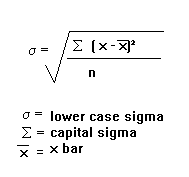Institutions: Global |ALU | Ekurhuleni Libraries | Joburg Libraries | Tshwane Libraries | TUT | UCT | UJ | UNISA | UP | UZ | Wits | Invest in Zim

MathsGee is Zero-Rated (You do not need data to access) on: Telkom |Dimension Data | Rain | MWEB

1 like 0 dislike
5,472 views
The variance of a sample of 169 observations equals 576. The standard deviation of the sample equals:
| 5,472 views

## 3 Answers

3 like 0 dislike
Best answer

The standard deviation is the measure of how the data differs from the mean. The equation is as follows:The equation inside the square root is the equation for the variance.

There for the answer is the 24.

by Wooden (588 points)
selected by
0 like 0 dislike
The standard deviation of the sample equals 24. The square root of 576.
by Wooden (476 points)
0 like 0 dislike
standard deviation is the squre root of a given sample space which is 24 at this case
by Wooden (424 points)

1 like 0 dislike
3 answers
3 like 1 dislike
5 answers
0 like 0 dislike
1 answer
0 like 0 dislike
0 answers
0 like 0 dislike
1 answer